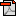SAT2 Math

SAT Mathematics Level 1 and Level 2 Format & Content

반응형getting-ready-for-the-sat-subj-tests.pdf

한글로 보기

 FORMAT 50 multiple-choice questions each Approximate Percentage of Test Topic Covered (topics in italics are tested on Level 2 Test only) Level 1 Test Level 2 Test Number and Operations 10% – 14% 10% – 14% Operations, ratio and proportion,  complex numbers, counting, elementary number theory, matrices, sequences, series, vectors Algebra and Functions 38% – 42% 48% – 52% Expressions, equations, inequalities, representation and modeling, properties of functions (linear, polynomial, rational, exponential, logarithmic, trigonometric, inverse trigonometric, periodic, piecewise, recursive, parametric) Geometry and Measurement 38% – 42% 28% – 32% Plane Euclidean/Measurement 18% – 22% – Coordinate 8% – 12% 10% – 14% Lines, parabolas, circles, ellipses, hyperbolas, symmetry, transformations, polar coordinates Three-dimensional 4% – 6% 4% – 6% Solids, surface area and volume (cylinders, cones, pyramids, spheres, prisms), coordinates in three dimensions Trigonometry 6% – 8% 12% – 16% Right triangles, identities, radian measure, law of cosines, law of sines, equations, double angle formulas Data Analysis, Statistics and Probability 8% – 12% 8% – 12% Mean, median, mode, range, interquartile range, standard deviation, graphs and plots, leastsquares regression (linear, quadratic, exponential), probability *The content of Level 1 overlaps somewhat with that on Level 2,    but the emphasis on Level 2 is on more advanced content.    Plane Euclidean geometry is not tested directly on Level 2.

Scores

Because the content measured by the two tests differs considerably, you cannot use your score on one test to predict your score on the other or to compare scores.

Calculators

Be sure to bring a calculator to use on these tests: If you take these tests without a calculator, you will be at a disadvantage. In fact, some questions cannot be solved without a scientific or a graphing calculator.

Verify that your calculator is in good working condition before you take the test.

If possible, bring batteries and a backup calculator to the test center. No substitute calculators or batteries will be available. Students may not share calculators.

If your calculator malfunctions during the Level 1 or Level 2 test and you do not have a backup calculator, you can cancel scores on just the mathematics test. You must tell your test supervisor when the malfunction occurs in order to cancel scores on these tests only.

Types of Calculators Recommended

Bring a calculator that you are used to using. It may be a scientific or a graphing calculator.

We recommend the use of a graphing calculator over a scientific calculator because a graphing calculator may provide an advantage on some questions.Do NOT Bring These Unacceptable Calculators

Models that have wireless, Bluetooth, cellular, audio/video recording and playing, camera, or any other smart phone type feature

Models that can access the Internet

Models that have QWERTY, pen-input, stylus,* or touch-screen capability; require electrical outlets; or use paper tape (e.g., TI-92 Plus, Voyage 200, Palm, PDAs, Casio ClassPad)

Models that “talk” or make unusual noises

*The use of the stylus with the Sharp EL-9600 calculator is not permitted.

Using the Calculator

You do not need to use a calculator to solve every question, and it is important to know when and how to use one. First decide how you will solve a problem; then determine whether the calculator is needed.

You’ll need a calculator for 40 to 50 percent of the questions on Level 1 and for 55 to 65 percent of the questions on Level 2.

For the rest of the questions, there is no advantage, perhaps even a disadvantage, to using a calculator.

Do not round any intermediate calculations. When you get a result from the calculator for the first step of a solution, keep the result in the calculator and use it for the second step. If you round the result from the first step, your answer may not be one of the choices.

You may not use a calculator for other Subject Tests and must put it away when not taking a mathematics test.

Geometric Figures

Figures that accompany problems are intended to provide information useful in solving the problems. They are drawn as accurately as possible EXCEPT when it is stated in a particular problem that the figure is not drawn to scale. Even when figures are not drawn to scale, the relative positions of points and angles may be assumed to be in the order shown. Also, line segments that extend through points and appear to lie on the same line may be assumed to be on the same line. The text “Note: Figure not drawn to scale” is included on the figure when degree measures may not be accurately shown and specific lengths may not be drawn proportionally.

반응형
사업자 정보 표시
EGO expert | 고봉기 | 서울 광진구 아차산로 345 래미안 프리미어팰리스 101동 2801호 | 사업자 등록번호 : 332-86-01007 | TEL : 010-2412-3152 | Mail : ego.expertgroup@gmail.com | 통신판매신고번호 : 2018-서울광진-0589호 | 사이버몰의 이용약관 바로가기

'SAT2 Math' 카테고리의 다른 글

 SAT Mathematics Level 1 and Level 2 Format & Content  (0) 2014.01.20 2014.01.20 2010.05.28 2010.04.26 2010.04.20 2010.04.19